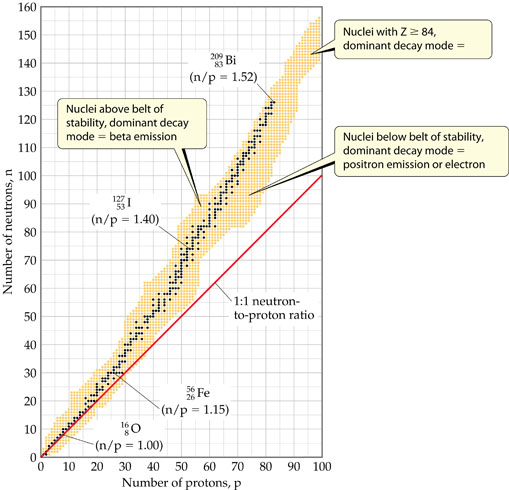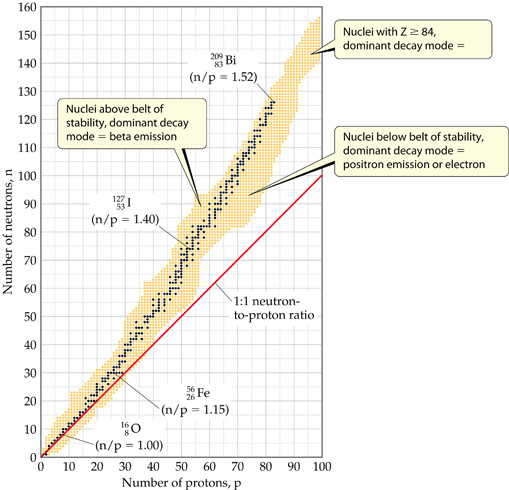# Problem: In 2010, a team of scientists from Russia and the U.S. reported the creation of the first atom of element 117, which is not yet named and is denoted . The synthesis involved the collision of a target of 97249Bk with accelerated ions of an isotope which we will denote Q. The product atom, which we will call Z, immediately releases neutrons and forms 117294:97249Bk  +  Q  →  Z  → 117294+ 301nIsotope Q is unusual in that it is very long-lived (its half-life is on the order of 1019 yr) in spite of having an unfavorable neutron-to-proton ratio. Can you propose a reason for its unusual stability?

###### FREE Expert Solution

We’re being asked to propose a reason for the unusual stability of isotope Q given the nuclear reaction:

${}_{\mathbf{97}}{}^{\mathbf{249}}\mathbf{Bk}\mathbf{+}\mathbf{Q}\mathbf{\to }\mathbf{Z}\mathbf{\to }{}_{\mathbf{117}}{}^{\mathbf{294}}\left[117\right]\mathbf{+}\mathbf{3}{}_{\mathbf{0}}{}^{\mathbf{1}}\mathbf{n}$

Recall that the stability of an isotope depends on the ratio of neutrons to protons (N/Z). This means for stable isotopes with:

• Z ≤ 20; the N/Z ratio should be equal to 1.0

• 20 < Z ≤ 40; the N/Z ratio should be equal to 1.25

• 40 < Z ≤ 80; the N/Z ratio should be equal to 1.50

• Z < 83; the stable nuclide does not exist

The N/Z values for stable isotopes form the valley or band of stability:We shall determine the identity of isotope Q:

We are to identify what Z is first:

Consider the part of the equation as follows:

$\mathbf{Z}\mathbf{\to }{}_{\mathbf{117}}{}^{\mathbf{294}}\mathbf{\left[}\mathbf{117}\mathbf{\right]}\mathbf{+}\mathbf{3}{}_{\mathbf{0}}{}^{\mathbf{1}}\mathbf{n}$

Recall:Step 1: Let’s first balance the atomic mass:

x = 294 + 3

x = 297 = atomic mass of the unknown isotope

Step 2: Next, we balance the atomic number:

x = 117+0

x = 117 = atomic number of the unknown istope

Step 3: The identity of Z is:

${}_{\mathbf{117}}{}^{\mathbf{297}}\mathbf{Z}$

We are to identify what Q is:

Consider the part of the equation as follows:

${}_{\mathbf{97}}{}^{\mathbf{249}}\mathbf{Bk}\mathbf{+}\mathbf{Q}\mathbf{\to }{}_{\mathbf{117}}{}^{\mathbf{297}}\mathbf{Z}$

Step 1: Let’s first balance the atomic mass:

249  + x =  297

x =297-249 = 48 = atomic mass of the unknown isotope

Step 2: Next, we balance the atomic number:

97 + x = 117

x =117-97 = 20 = atomic number of the unknown istope

Step 3: The identity of Q (i.e. the atomic number determines the identity of the element) is:

${}_{\mathbf{20}}{}^{\mathbf{48}}\mathbf{Ca}$

92% (403 ratings)###### Problem Details

In 2010, a team of scientists from Russia and the U.S. reported the creation of the first atom of element 117, which is not yet named and is denoted . The synthesis involved the collision of a target of 97249Bk with accelerated ions of an isotope which we will denote Q. The product atom, which we will call Z, immediately releases neutrons and forms 117294:
97249Bk  +  Q  →  Z  → 117294+ 301n

Isotope Q is unusual in that it is very long-lived (its half-life is on the order of 1019 yr) in spite of having an unfavorable neutron-to-proton ratio.Can you propose a reason for its unusual stability?